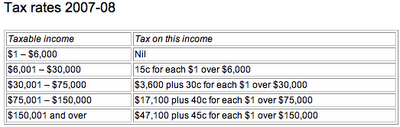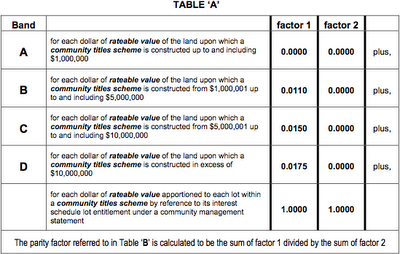## Saturday, 28 June 2008

### The Great Brisbane Rates Kerfuffle

The Brisbane city council's 2008 budget has resulted in widespread angst and anguish over a proposed change in the property rates formula for units (apartments on community titles, what the Americans call condos). The proposal is to add a parity factor, an Orwellian name if ever there was one, to ensure that some people pay on a higher scale.

The issue as I understand it is that dwellings are assessed on the basis of the rateable value of their land, and not the building. This formula may in some cases mean that an apartment is rated less than a house of equivalent value. For example, an upmarket penthouse which is 1% of the area of a large unit block on land worth \$40-million may be rated at a value of \$400,000 – despite being worth several million dollars.

Applying this formula can therefore an result in rateable values not equivalent in comparison with market value. The Brisbane Lord Mayor Campbell Newman (this is what they call mayors of big cities here, after the UK practice) obviously felt that this was inequitable, citing examples of million-dollar penthouses being assessed at a lower rate than houses in the outer suburbs that sell for much less. The obvious fix would be to change the valuation formula so that it correctly reflected the true resale value.

Absent that, the approach in the latest budget has been to add in a "parity factor" that increases the rent of units on higher-valued land. The problem is that this formula does not take into account the size of the land, or the number of units. This, a smallish \$20-million block of land with a small number of luxury apartments on it has the same multiplier as a largish block of land of the same value, with hundreds of apartments on it. Worse, if you have multiple buildings on a single block of land, the exact same configuration of buildings would have a lower multiplier if the land were subdivided. To take an extreme case, if 10 buildings were on a block of land worth \$10-million and the land was not divided, they would attract a multiplier near the top of the scale. If that land had instead been divided with one building per subdivision and each block of land was now worth \$1-million, the multiplier would be zero.

Some ratepayers have found that this new formula results in increases of the order of 700%.

Or does it?

Others interpreting the formula, including city council employees and opposition councillors, have interpreted the table defining the formula as having the same effect as something like an income tax table. It's worth quoting the text of the current Australian Tax Office income tax table here for comparison.The key thing to note here is the wording in each category, which defines the amount to accumulate to the total, e.g., "\$3,600 plus 30c for each \$1 over \$30,000". Remember the important bit: so many cents for each \$1 over the current threshold.

Right, now let's look at the table in the budget papers. Look for page 290 (or page 18 of the PDF document).Observe here the crucial difference in the wording. Each entry in the table under the heading "factor 1" is just a number, a small fraction. It is not so many cents in the dollar.

Let's look at how this formula, if applied exactly as specified in the table, applies in the case where your unit is on land valued at \$20-million. Each row of the table says something like for the following portion of the value of the property, factor 1 is x, and factor 2 is y. Factor 2 is 0 in all cases but one, so let's focus on factor 1.

• Band A of the table says you add 0 on for the first \$1-million: so for, 0
• Band B of the table says you add 0.0110 on for the next \$4-million: that's 0.0110
• Band C of the table says you add 0.0150 on for the next \$5-million: another 0.0150
• Band D of the table says you add 0.0175 on for anything over \$10-million: another 0.0175

Next step: how do we combine all these values? The difference of opinion comes in the wording in the table saying "for each dollar of rateable value of the land upon which a community titles scheme is constructed from \$1,000,001 up to and including \$5,000,000" (to take Band B as an example). My interpretation of this is that the relevant factor 1 should be weighted according to the fraction of the purchase price that is covered by this band. For example, for our hypothetical \$20-million property, Band B represents a quarter of its value. Doing the same for all the bands results in the following:

• Band A: 0
• Band B: 0.0110 times 0.2 (\$4-million is a fifth of the value); that's 0.0022
• Band C: 0.0150 times 0.25 (\$5-million is a quarter of the value); that's 0.00375
• Band D: 0.0175 times 0.5 (the value over \$10-million is 50% of the total): another 0.00875

So the overall total of Bands A+B+C+D should be 0 + 0.0022 + 0.00375 + 0.00875 = 0.0147. to complete the formula, we must add 1, then divide by factor 2, which is also 1, resulting in a final calculation of 1.0147.

In other words, the increase over the normal general rates calculation is 1.47%.

Is this right?

I argue that the interpretation others are placing on the formula requires that it be rewritten (taking Band B as an example) to read something like this:

1.1c for each dollar of rateable value of the land upon which a community titles scheme is constructed from \$1,000,001 up to and including \$5,000,000

Without this wording to read it the way I did is perfectly reasonable. To read it otherwise requires an interpretation that is not actually in words in the budget papers. The description of the calculation clearly tells you to that "The parity factor referred to in Table 'B' is calculated to be the sum of factor 1 divided by the sum of factor 2" – and the table has a heading of "factor 1" over the fractions, and not over the descriptive text describing the bands. It is therefore a stretch to say that "factor 1" is calculated as the given fraction times the dollar amount represented by the band. There is nothing in the text suggesting you should do so. It is in fact not entirely clear that the weighted average that I calculated is correct either, but that is the best interpretation I can put on this document.

The conclusion?

The city council should not have made this change without consultation. Not only has it had unintended consequences of penalising people who are not wealthy in the intended form, but the wording in the budget papers is open to another interpretation with a radically different effect.

I'm not a lawyer or an accountant; I would be curious to see if the council's interpretation would stand up in court.